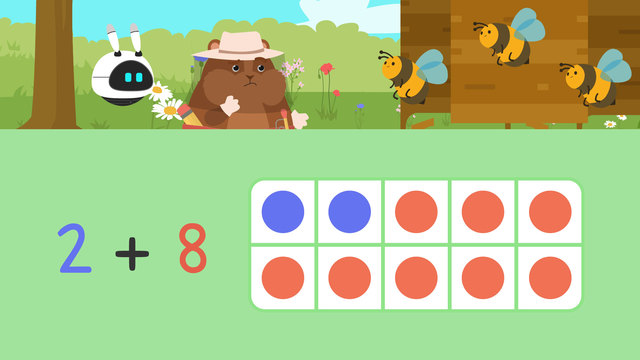# Number Pairs to 10Rate this video

Ø 5.0 / 2 ratings

The authorTeam Digital
Number Pairs to 10
CCSS.MATH.CONTENT.1.OA.C.6

## Information about the videoNumber Pairs to 10

### In This Video on Number Pairs

The local bees are trying to figure out how many more bees can fit into each hive to make ten, but need Mr. Squeaks’ help to figure out how many more can fit! Mr. Squeaks saves the day by using number pairs to 10 to solve the problem! After this video, there is a number pairs to 10 worksheet for further practice.

### Group Pairs of Successive Numbers that Add to 10

There are nine different pairs of numbers that add together to make ten. They are one plus nine, two plus eight, three plus seven, four plus six, five plus five, six plus four, seven plus three, eight plus two, and nine plus one!### Finding Number Pairs to Ten Example

Let’s imagine that one of the hives already has four bees inside! We can use a tens frame to set this up with four counters.To find the missing number, we can count the empty spaces on the tens frame to find the missing number pair for four that makes ten! There are six empty spaces, so the missing number pair to make ten here is six!### Number Pairs to 10 Review

Remember, there are nine pairs of numbers that make ten. However, you only have to memorize five of them because some are the same reversed. For example, one plus nine is the same as nine plus one!Below is a finding pairs of numbers that add to 10 worksheet.

### TranscriptNumber Pairs to 10

"Hey! What's all the buzzing about here?" It appears the bees love to be in groups of ten in their hives, but they need help figuring out how many more bees are needed for each hive! Let's help Mr. Squeaks sort the bees into groups of ten by learning about number pairs to ten. Number pairs to ten are two numbers added together to make ten! A tens frame like this can help us to make ten. There are nine different pairs of numbers that add together to make ten. They are; one plus nine, two plus eight, three plus seven, four plus six, five plus five, six plus four, seven plus three, eight plus two, and nine plus one. However, you only need to remember five pairs of numbers.All pairs, except five plus five, can have their order changed, or reversed, to make the other pair. One plus nine is the same as nine plus one. Two plus eight is the same as eight plus two. What is the same as three plus seven? Three plus seven is the same as seven plus three. What is the same as four plus six? Four plus six is the same as six plus four. Now we are ready to help sort the bees into hives of ten. The first hive already has four bees inside it. The tens frame has four counters to show this. We can count the empty spaces to find the missing number pair! Let's count; one, two, three, four, five, six! Six is the missing number. Four plus six make ten. The next hive already has five bees inside it. The tens frame has five counters to show this. Again, count the empty spaces. How many empty spaces are there? One, two, three, four, five! Five is the missing number. Five plus five make ten. The final hive already has three bees inside it. What is the missing number pair to make ten here? Seven is the missing number. Three plus seven make ten. While the bees head to their hives, let's review! Remember, today we learned there are nine pairs of numbers that make ten. They are; one plus nine, two plus eight, three plus seven, four plus six, five plus five, six plus four, seven plus three, eight plus two, and nine plus one. "Erm, Imani, what are you doing?"